The development of big data platforms allows you to process multiple types of unstructured and semi-structured data. For example, you can convert IP addresses into geolocations. This topic describes how to use a MaxCompute user-defined function (UDF) to convert IPv4 or IPv6 addresses into geolocations.

Prerequisites

Make sure that the following requirements are met:

Background information

To convert IPv4 or IPv6 addresses into geolocations, you must download the IP address library file that includes the IP addresses, and upload the file to the MaxCompute project as a resource. After you develop and create a MaxCompute UDF based on the IP address library file, you can call the UDF in SQL statements to convert IP addresses into geolocations.

Usage notes

The IP address library file provided in this topic is for reference only. You must maintain the IP address library file based on your business requirements.

Procedure

To convert IPv4 or IPv6 addresses into geolocations by using a MaxCompute UDF, perform the following steps:

Upload an IP address library file as a resource to your MaxCompute project. The resource is used when you create a MaxCompute UDF in subsequent steps.
2. Step 2: Write a MaxCompute UDF
Write a MaxCompute UDF by using IntelliJ IDEA.
3. Step 3: Create the MaxCompute UDF
Create the MaxCompute UDF.
4. Step 4: Call the MaxCompute UDF to convert an IP address into a geolocation
Call the MaxCompute UDF that you created in an SQL statement to convert IP addresses into geolocations.

1. Download an IP address library file to your on-premise machine, decompress the file to obtain the ipv4.txt and ipv6.txt files, and then place the files in the installation directory of the MaxCompute client, ...\odpscmd_public\bin.

The IP address library file provided in this topic is for reference only. You must maintain the IP address library file based on your business requirements.

2. Start the MaxCompute client and go to the MaxCompute project to which you want to upload the ipv4.txt and ipv6.txt files.
3. Run the add file command to upload the two files as file resources to the MaxCompute project.
Sample commands:

Step 2: Write a MaxCompute UDF

1. Create a Java class.
The Java class is used for writing a MaxCompute UDF in the next substep.
1. Start IntelliJ IDEA. In the left-side navigation pane of the Project tab, choose src > main > java, right-click java, and then choose New > Java Class.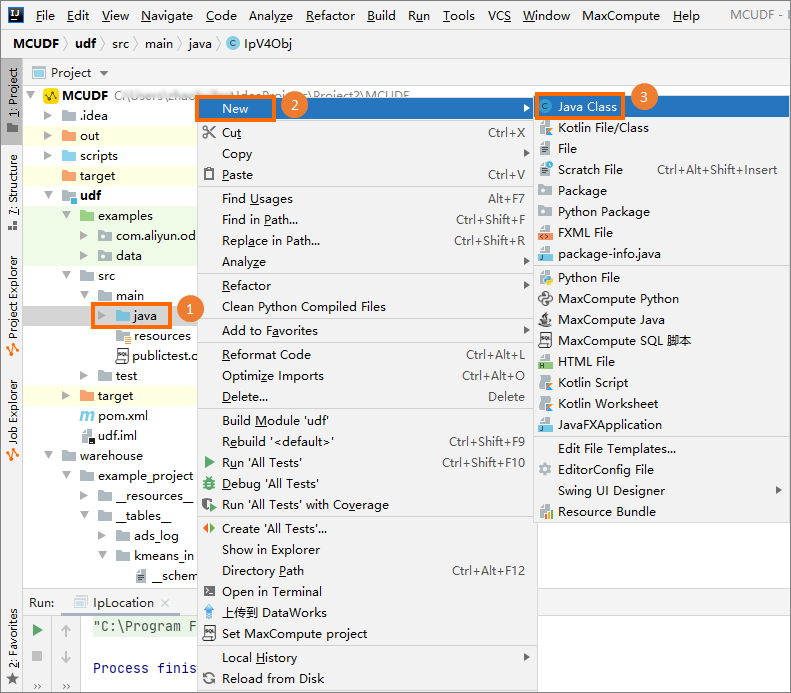2. In the New Java Class dialog box, enter a class name, press Enter, and then enter the code in the code editor.
You must create three Java classes. The following sections show the names and code of these classes. You can reuse the code without modification.
• IpUtils
package com.aliyun.odps.udf.utils;

import java.math.BigInteger;
import java.net.UnknownHostException;
import java.util.Arrays;

public class IpUtils {

/**
* Convert the data type of IP addresses from STRING to LONG.
*
* @param ipInString
* IP addresses of the STRING type.
* @return Return the IP addresses of the LONG type.
*/
public static long StringToLong(String ipInString) {

ipInString = ipInString.replace(" ", "");
byte[] bytes;
if (ipInString.contains(":"))
bytes = ipv6ToBytes(ipInString);
else
bytes = ipv4ToBytes(ipInString);
BigInteger bigInt = new BigInteger(bytes);
//        System.out.println(bigInt.toString());
return bigInt.longValue();
}

/**
* Convert the data type of IP addresses from STRING to LONG.
*
* @param ipInString
* IP addresses of the STRING type.
* @return Return the IP addresses of the STRING type that is converted from BIGINT.
*/
public static String StringToBigIntString(String ipInString) {

ipInString = ipInString.replace(" ", "");
byte[] bytes;
if (ipInString.contains(":"))
bytes = ipv6ToBytes(ipInString);
else
bytes = ipv4ToBytes(ipInString);
BigInteger bigInt = new BigInteger(bytes);
return bigInt.toString();
}

/**
* Convert the data type of IP addresses from BIGINT to STRING.
*
* @param ipInBigInt
* IP addresses of the BIGINT type.
* @return Return the IP addresses of the STRING type.
*/
public static String BigIntToString(BigInteger ipInBigInt) {
byte[] bytes = ipInBigInt.toByteArray();
byte[] unsignedBytes = Arrays.copyOfRange(bytes, 1, bytes.length);
// Remove the sign bit.
try {
return ip.substring(ip.indexOf('/') + 1).trim();
} catch (UnknownHostException e) {
throw new RuntimeException(e);
}
}

/**
* Convert the data type of IPv6 addresses into signed byte 17.
*/
private static byte[] ipv6ToBytes(String ipv6) {
byte[] ret = new byte;
ret = 0;
int ib = 16;
boolean comFlag=false;// IPv4/IPv6 flag.
if (ipv6.startsWith(":"))// Remove the colon (:) from the start of IPv6 addresses.
ipv6 = ipv6.substring(1);
String groups[] = ipv6.split(":");
for (int ig=groups.length - 1; ig > -1; ig--) {// Reverse scan.
if (groups[ig].contains(".")) {
// Both IPv4 and IPv6 addresses exist.
byte[] temp = ipv4ToBytes(groups[ig]);
ret[ib--] = temp;
ret[ib--] = temp;
ret[ib--] = temp;
ret[ib--] = temp;
comFlag = true;
} else if ("".equals(groups[ig])) {
// Zero-length compression. Calculate the number of missing groups.
int zlg = 9 - (groups.length + (comFlag ? 1 : 0));
while (zlg-- > 0) {// Set these groups to 0.
ret[ib--] = 0;
ret[ib--] = 0;
}
} else {
int temp = Integer.parseInt(groups[ig], 16);
ret[ib--] = (byte) temp;
ret[ib--] = (byte) (temp >> 8);
}
}
return ret;
}

/**
* Convert the data type of IPv4 addresses into signed byte 5.
*/
private static byte[] ipv4ToBytes(String ipv4) {
byte[] ret = new byte;
ret = 0;
// Find the positions of the periods (.) in the IP addresses of the STRING type.
int position1 = ipv4.indexOf(".");
int position2 = ipv4.indexOf(".", position1 + 1);
int position3 = ipv4.indexOf(".", position2 + 1);
// Convert the IP addresses of the STRING type between periods (.) into INTEGER.
ret = (byte) Integer.parseInt(ipv4.substring(0, position1));
ret = (byte) Integer.parseInt(ipv4.substring(position1 + 1,
position2));
ret = (byte) Integer.parseInt(ipv4.substring(position2 + 1,
position3));
ret = (byte) Integer.parseInt(ipv4.substring(position3 + 1));
return ret;
}

/**
* @return 4:IPv4, 6:IPv6, 0: Invalid IP addresses.
* @throws Exception
*/
public static int isIpV4OrV6(String ipAdress) throws Exception {
return 4;
return 6;
return 0;
}

/*
* Check whether the IP address belongs to a specific IP section.
*
* ipSection The IP sections that are separated by hyphens (-).
*
* The IP address to check.
*/

public static boolean ipExistsInRange(String ip, String ipSection) {

ipSection = ipSection.trim();

ip = ip.trim();

int idx = ipSection.indexOf('-');

String beginIP = ipSection.substring(0, idx);

String endIP = ipSection.substring(idx + 1);

return getIp2long(beginIP) <= getIp2long(ip)
&& getIp2long(ip) <= getIp2long(endIP);

}

public static long getIp2long(String ip) {

ip = ip.trim();

String[] ips = ip.split("\\.");

long ip2long = 0L;

for (int i = 0; i < 4; ++i) {

ip2long = ip2long << 8 | Integer.parseInt(ips[i]);

}
return ip2long;

}

public static long getIp2long2(String ip) {

ip = ip.trim();

String[] ips = ip.split("\\.");

long ip1 = Integer.parseInt(ips);

long ip2 = Integer.parseInt(ips);

long ip3 = Integer.parseInt(ips);

long ip4 = Integer.parseInt(ips);

long ip2long = 1L * ip1 * 256 * 256 * 256 + ip2 * 256 * 256 + ip3 * 256
+ ip4;

return ip2long;

}

public static void main(String[] args) {
System.out.println(StringToLong("2002:7af3:f3be:ffff:ffff:ffff:ffff:ffff"));
System.out.println(StringToLong("54.38.72.63"));
}

private class Invalid{
private Invalid()
{

}
}
}

• IpV4Obj
package com.aliyun.odps.udf.objects;

public class IpV4Obj {
public long startIp ;
public long endIp ;
public String city;
public String province;

public IpV4Obj(long startIp, long endIp, String city, String province) {
this.startIp = startIp;
this.endIp = endIp;
this.city = city;
this.province = province;
}

@Override
public String toString() {
return "IpV4Obj{" +
"startIp=" + startIp +
", endIp=" + endIp +
", city='" + city + '\'' +
", province='" + province + '\'' +
'}';
}

public void setStartIp(long startIp) {
this.startIp = startIp;
}

public void setEndIp(long endIp) {
this.endIp = endIp;
}

public void setCity(String city) {
this.city = city;
}

public void setProvince(String province) {
this.province = province;
}

public long getStartIp() {
return startIp;
}

public long getEndIp() {
return endIp;
}

public String getCity() {
return city;
}

public String getProvince() {
return province;
}
}

• IpV6Obj
package com.aliyun.odps.udf.objects;

public class IpV6Obj {
public String startIp ;
public String endIp ;
public String city;
public String province;

public String getStartIp() {
return startIp;
}

@Override
public String toString() {
return "IpV6Obj{" +
"startIp='" + startIp + '\'' +
", endIp='" + endIp + '\'' +
", city='" + city + '\'' +
", province='" + province + '\'' +
'}';
}

public IpV6Obj(String startIp, String endIp, String city, String province) {
this.startIp = startIp;
this.endIp = endIp;
this.city = city;
this.province = province;
}

public void setStartIp(String startIp) {
this.startIp = startIp;
}

public String getEndIp() {
return endIp;
}

public void setEndIp(String endIp) {
this.endIp = endIp;
}

public String getCity() {
return city;
}

public void setCity(String city) {
this.city = city;
}

public String getProvince() {
return province;
}

public void setProvince(String province) {
this.province = province;
}
}

2. Write a MaxCompute UDF.
1. In the left-side navigation pane of the Project tab, choose src > main > java, right-click java, and then choose New > MaxCompute Java.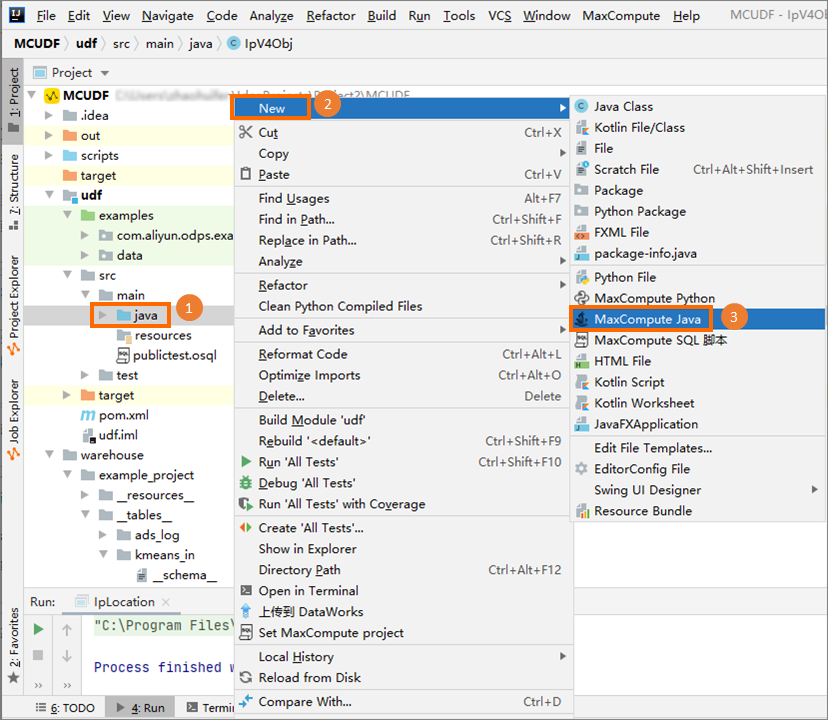2. In the Create new MaxCompute java class dialog box, click UDF and enter a class name in the Name field. Then, press Enter and enter the code in the code editor.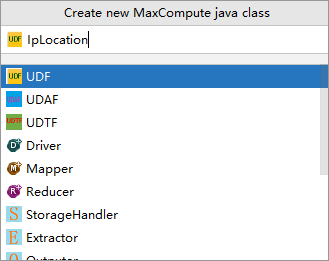The following code shows how to write a UDF based on a Java class named IpLocation. You can reuse the code without modification.
package com.aliyun.odps.udf.udfFunction;

import com.aliyun.odps.udf.ExecutionContext;
import com.aliyun.odps.udf.UDF;
import com.aliyun.odps.udf.UDFException;
import com.aliyun.odps.udf.utils.IpUtils;
import com.aliyun.odps.udf.objects.IpV4Obj;
import com.aliyun.odps.udf.objects.IpV6Obj;
import java.io.*;
import java.util.ArrayList;
import java.util.Comparator;
import java.util.List;
import java.util.stream.Collectors;

public class IpLocation extends UDF {
public static IpV4Obj[] ipV4ObjsArray;
public static IpV6Obj[] ipV6ObjsArray;

public IpLocation() {
super();
}

@Override
public void setup(ExecutionContext ctx) throws UDFException, IOException {
//IPV4
if(ipV4ObjsArray==null)
{

ArrayList<IpV4Obj> ipV4ObjArrayList=new ArrayList<>();
String line = null;
while ((line = br.readLine()) != null) {
String[] f = line.split("\\|", -1);
if(f.length>=5)
{
long startIp = IpUtils.StringToLong(f);
long endIp = IpUtils.StringToLong(f);
String city=f;
String province=f;
IpV4Obj ipV4Obj = new IpV4Obj(startIp, endIp, city, province);
}
}
br.close();
List<IpV4Obj> collect = ipV4ObjArrayList.stream().sorted(Comparator.comparing(IpV4Obj::getStartIp)).collect(Collectors.toList());
ArrayList<IpV4Obj> basicIpV4DataList=(ArrayList)collect;
IpV4Obj[] ipV4Objs = new IpV4Obj[basicIpV4DataList.size()];
ipV4ObjsArray = basicIpV4DataList.toArray(ipV4Objs);
}

//IPV6
if(ipV6ObjsArray==null)
{
ArrayList<IpV6Obj> ipV6ObjArrayList=new ArrayList<>();
String line = null;
while ((line = br.readLine()) != null) {
String[] f = line.split("\\|", -1);
if(f.length>=5)
{
String startIp = IpUtils.StringToBigIntString(f);
String endIp = IpUtils.StringToBigIntString(f);
String city=f;
String province=f;
IpV6Obj ipV6Obj = new IpV6Obj(startIp, endIp, city, province);
}
}
br.close();
List<IpV6Obj> collect = ipV6ObjArrayList.stream().sorted(Comparator.comparing(IpV6Obj::getStartIp)).collect(Collectors.toList());
ArrayList<IpV6Obj> basicIpV6DataList=(ArrayList)collect;
IpV6Obj[] ipV6Objs = new IpV6Obj[basicIpV6DataList.size()];
ipV6ObjsArray = basicIpV6DataList.toArray(ipV6Objs);
}

}

public String evaluate(String ip){
if(ip==null||ip.trim().isEmpty()||!(ip.contains(".")||ip.contains(":")))
{
return null;
}
int ipV4OrV6=0;
try {
ipV4OrV6= IpUtils.isIpV4OrV6(ip);
} catch (Exception e) {
return null;
}
if(ipV4OrV6==4)
{
int i = binarySearch(ipV4ObjsArray, IpUtils.StringToLong(ip));
if(i>=0)
{
IpV4Obj ipV4Obj = ipV4ObjsArray[i];
return ipV4Obj.city+","+ipV4Obj.province;
}else{
return null;
}
} else if(ipV4OrV6==6)// IPv6 addresses are used.
{
int i = binarySearchIPV6(ipV6ObjsArray, IpUtils.StringToBigIntString(ip));
if(i>=0)
{
IpV6Obj ipV6Obj = ipV6ObjsArray[i];
return ipV6Obj.city+","+ipV6Obj.province;
}else{
return null;
}
} else{// IP addresses are invalid.
return null;
}

}

@Override
public void close() throws UDFException, IOException {
super.close();
}

private static int binarySearch(IpV4Obj[] array,long ip){
int low=0;
int hight=array.length-1;
while (low<=hight)
{
int middle=(low+hight)/2;
if((ip>=array[middle].startIp)&&(ip<=array[middle].endIp))
{
return middle;
}
if (ip < array[middle].startIp)
hight = middle - 1;
else {
low = middle + 1;
}
}
return -1;
}

private static int binarySearchIPV6(IpV6Obj[] array,String ip){
int low=0;
int hight=array.length-1;
while (low<=hight)
{
int middle=(low+hight)/2;
if((ip.compareTo(array[middle].startIp)>=0)&&(ip.compareTo(array[middle].endIp)<=0))
{
return middle;
}
if (ip.compareTo(array[middle].startIp) < 0)
hight = middle - 1;
else {
low = middle + 1;
}
}
return -1;
}

private class Invalid{
private Invalid()
{

}
}
}

3. Debug the MaxCompute UDF to check whether the code is run as expected.
For more information about how to debug UDFs, see Perform a local run to debug the UDF.
1. Right-click the MaxCompute UDF script that you wrote and select Run.
2. In the Run/Debug Configurations dialog box, configure the required parameters and click OK, as shown in the following figure.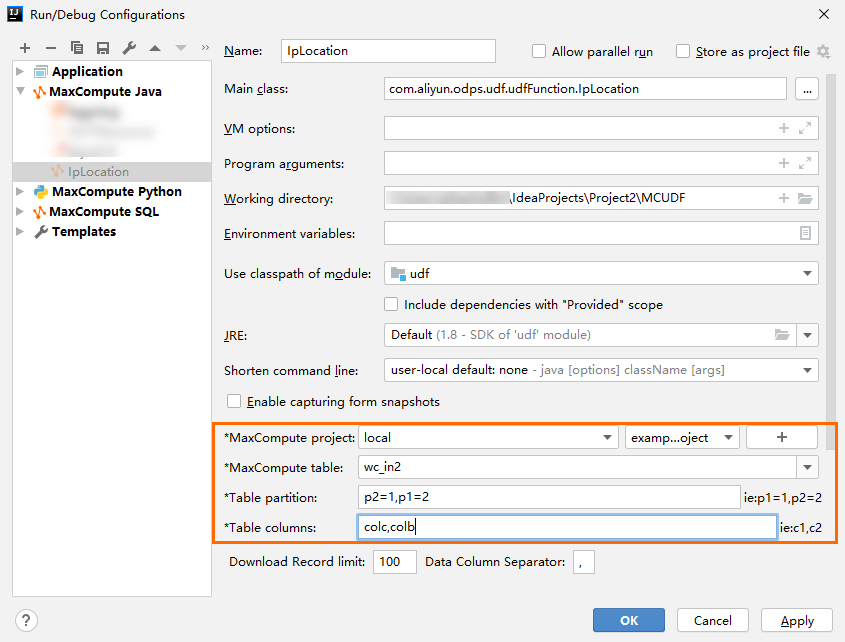If no error is returned, the code is run successfully. You can proceed with subsequent steps. If an error is reported, you can perform troubleshooting based on the error information displayed on IntelliJ IDEA.
Note The parameter settings in the preceding figure are provided for reference.

Step 3: Create the MaxCompute UDF

1. Right-click the MaxCompute UDF script that you compiled and select Deploy to server….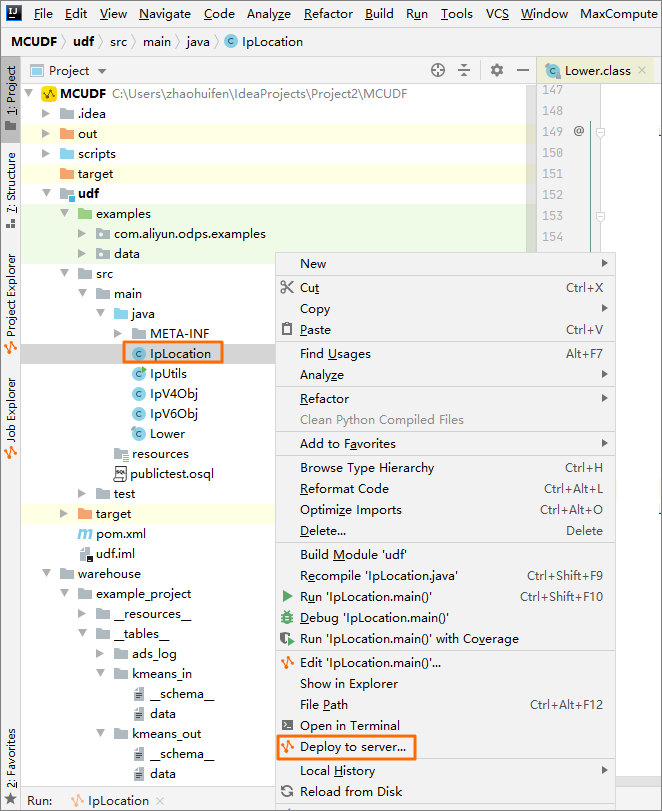2. In the Package a jar, submit resource and register function dialog box, configure the parameters.
For more information about the parameters, see Package, upload, and register a Java program.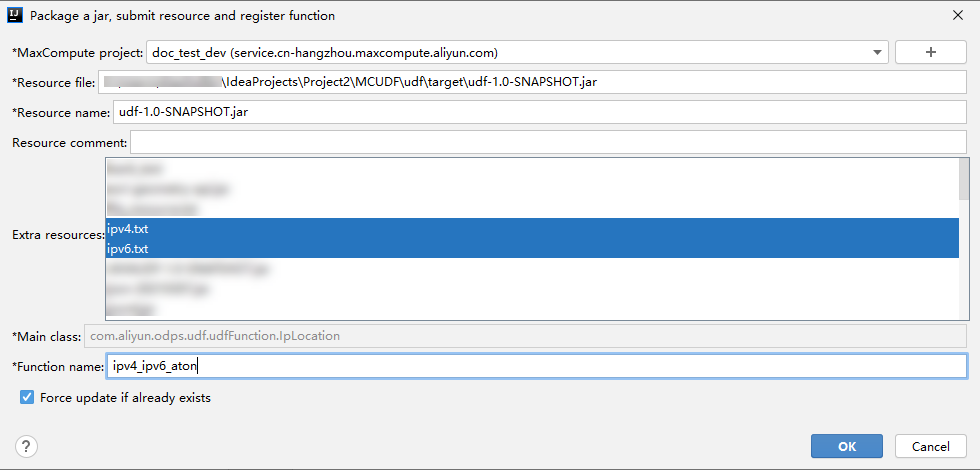Extra resources: You must select the IP address library files ipv4.txt and ipv6.txt that you uploaded in Step 1. In this topic, the created function is named ipv4_ipv6_aton.

Step 4: Call the MaxCompute UDF to convert an IP address into a geolocation

1. You can execute an SQL SELECT statement to call the MaxCompute UDF to convert an IPv4 or IPv6 address into a geolocation.
Sample statements:
• Convert an IPv4 address into a geolocation
select ipv4_ipv6_aton('116.11.34.15');
The following result is returned:
Beihai, Guangxi Zhuang Autonomous Region
• Convert an IPv6 address into a geolocation
select ipv4_ipv6_aton('2001:0250:080b:0:0:0:0:0');
The following result is returned:
Baoding, Hebei Province